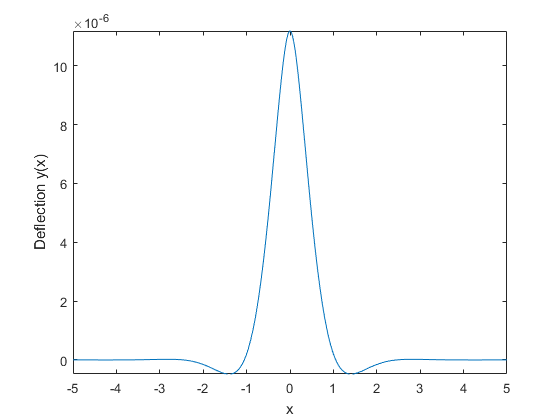Documentation

## Fourier and Inverse Fourier Transforms

This page shows the workflow for Fourier and inverse Fourier transforms in Symbolic Math Toolbox™. For simple examples, see `fourier` and `ifourier`. Here, the workflow for Fourier transforms is demonstrated by calculating the deflection of a beam due to a force. The associated differential equation is solved by the Fourier transform.

### Fourier Transform Definition

The Fourier transform of f(x) with respect to x at w is

`$F\left(w\right)=\underset{-\infty }{\overset{\infty }{\int }}f\left(x\right){e}^{-iwx}dx.$`

The inverse Fourier transform is

`$f\left(x\right)=\frac{1}{2\pi }\underset{-\infty }{\overset{\infty }{\int }}F\left(w\right){e}^{iwx}dw.$`

### Concept: Using Symbolic Workflows

Symbolic workflows keep calculations in the natural symbolic form instead of numeric form. This approach helps you understand the properties of your solution and use exact symbolic values. You substitute numbers in place of symbolic variables only when you require a numeric result or you cannot continue symbolically. For details, see Choose Symbolic or Numeric Arithmetic. Typically, the steps are:

1. Declare equations.

2. Solve equations.

3. Substitute values.

4. Plot results.

5. Analyze results.

### Calculate Beam Deflection Using Fourier Transform

#### Define Equations

Fourier transform can be used to solve ordinary and partial differential equations. For example, you can model the deflection of an infinitely long beam resting on an elastic foundation under a point force. A corresponding real-world example is railway tracks on a foundation. The railway tracks are the infinitely long beam while the foundation is elastic.Let

• E be the elasticity of the beam (or railway track).

• I be the second moment of area of the cross-section of the beam.

• k be the spring stiffness of the foundation.

The differential equation is

Define the function `y(x)` and the variables. Assume `E`, `I`, and `k` are positive.

```syms Y(x) w E I k f assume([E I k] > 0)```

Assign units to the variables by using `symunit`.

```u = symunit; Eu = E*u.Pa; % Pascal Iu = I*u.m^4; % meter^4 ku = k*u.N/u.m^2; % Newton/meter^2 X = x*u.m; F = f*u.N/u.m;```

Define the differential equation.

`eqn = diff(Y,X,4) + ku/(Eu*Iu)*Y == F/(Eu*Iu)`
```eqn(x) = diff(Y(x), x, x, x, x)*(1/[m]^4) + ((k*Y(x))/(E*I))*([N]/([Pa]*[m]^6)) == ... (f/(E*I))*([N]/([Pa]*[m]^5))```

Represent the force `f` by the Dirac delta function δ(x).

`eqn = subs(eqn,f,dirac(x))`
```eqn(x) = diff(Y(x), x, x, x, x)*(1/[m]^4) + ((k*Y(x))/(E*I))*([N]/([Pa]*[m]^6)) ==... (dirac(x)/(E*I))*([N]/([Pa]*[m]^5))```

#### Solve Equations

Calculate the Fourier transform of `eqn` by using `fourier` on both sides of `eqn`. The Fourier transform converts differentiation into exponents of `w`.

`eqnFT = fourier(lhs(eqn)) == fourier(rhs(eqn))`
```eqnFT = w^4*fourier(Y(x), x, w)*(1/[m]^4) + ((k*fourier(Y(x), x, w))/(E*I))*([N]/([Pa]*[m]^6)) == (1/(E*I))*([N]/([Pa]*[m]^5))```

Isolate `fourier(Y(x),x,w)` in the equation.

`eqnFT = isolate(eqnFT, fourier(Y(x),x,w))`
```eqnFT = fourier(Y(x), x, w) == (1/(E*I*w^4*[Pa]*[m]^2 + k*[N]))*[N]*[m]```

Calculate `Y(x)` by calculating the inverse Fourier transform of the right side. Simplify the result.

```YSol = ifourier(rhs(eqnFT)); YSol = simplify(YSol)```
```YSol = ((exp(-(2^(1/2)*k^(1/4)*abs(x))/(2*E^(1/4)*I^(1/4)))*sin((2*2^(1/2)*k^(1/4)*abs(x) +... pi*E^(1/4)*I^(1/4))/(4*E^(1/4)*I^(1/4))))/(2*E^(1/4)*I^(1/4)*k^(3/4)))*[m]```

Check that `YSol` has the correct dimensions by substituting `YSol` into `eqn` and using the `checkUnits` function. `checkUnits` returns logical `1` (`true`), meaning `eqn` now has compatible units of the same physical dimensions.

`checkUnits(subs(eqn,Y,YSol))`
```ans = struct with fields: Consistent: 1 Compatible: 1```

Separate the expression from the units by using `separateUnits`.

`YSol = separateUnits(YSol)`
```YSol = (exp(-(2^(1/2)*k^(1/4)*abs(x))/(2*E^(1/4)*I^(1/4)))*sin((2*2^(1/2)*k^(1/4)*abs(x) + pi*E^(1/4)*I^(1/4))/(4*E^(1/4)*I^(1/4))))/(2*E^(1/4)*I^(1/4)*k^(3/4))```

#### Substitute Values

Use the values E = 106 Pa, I = 10-3 m4, and k = 106 N/m2. Substitute these values into `YSol` and convert to floating point by using `vpa` with 16 digits of accuracy.

```values = [1e6 1e-3 1e5]; YSol = subs(YSol,[E I k],values); YSol = vpa(YSol,16)```
```YSol = 0.0000158113883008419*exp(-2.23606797749979*abs(x))*sin(2.23606797749979*abs(x) + 0.7853981633974483)```

#### Plot Results

Plot the result by using `fplot`.

```fplot(YSol) xlabel('x') ylabel('Deflection y(x)')```
``

#### Analyze Results

The plot shows that the deflection of a beam due to a point force is highly localized. The deflection is greatest at the point of impact and then decreases quickly. The symbolic result enables you to analyze the properties of the result, which is not possible with numeric results.

Notice that `YSol` is a product of terms. The term with `sin` shows that the response is vibrating oscillatory behavior. The term with `exp` shows that the oscillatory behavior is quickly damped by the exponential decay as the distance from the point of impact increases.

#### Mathematical Modeling with Symbolic Math Toolbox

Get examples and videos